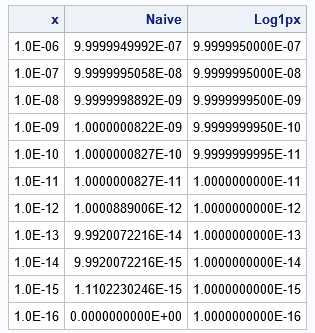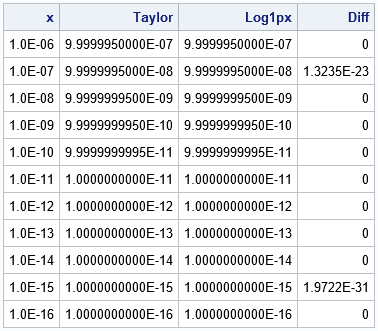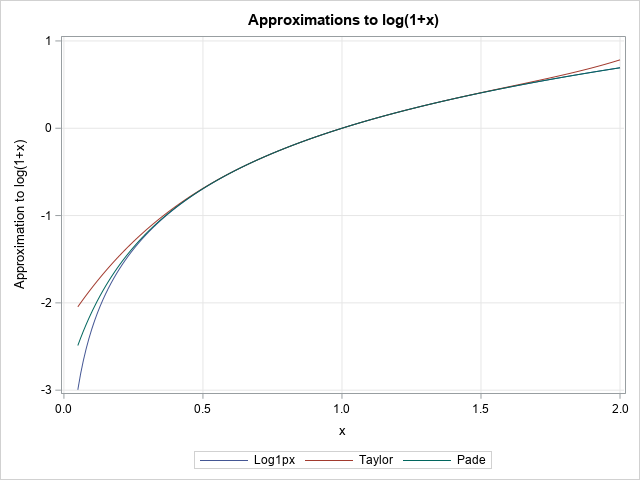SAS supports a special function for the accurate evaluation of log(1+x) when x is near 0. The LOG1PX function is useful because a naive computation of log(1+x) loses accuracy when x is near 0. This article demonstrates two general approximation techniques that are often used in numerical analysis: the Taylor series expansion and the approximation by a rational function. A rational function is a ratio of two polynomials.

### Understanding the problem

In numerical computations, expressions of the form 1 + x can be inaccurate when x is very small in magnitude. If x is smaller than machine precision (also called machine epsilon), then the expression 1 + x is exactly equal to 1. In SAS and other double-precision numerical software, machine precision is about 2.22E-16. In SAS, you can use the constant function to find the value of machine epsilon and other constants.

Even for moderate values of x, the expression 1 + x loses precision, which means that functions like log(1 + x) also lose precision. In numerical computations, it is helpful to know what answer to expect. For log(1+x), the Taylor series near x=0 is log(1+x) = x - x2/2 + O(x3), which means that the correct answer should be slightly less than x when x is a small positive value. Let's compute the expression log(1 + x) in two ways: by naively calling log(1+x) and by calling the special function LOG1PX. For very small positive values of x, we expect the first expression to lose precision but the second to be accurate.

```data log1px; do pow = -6 to -16 by -1; x = 10**pow; Naive = log(1+x); /* naive computation: loses accuracy */ Log1px = log1px(x); /* special function: more accurate */ output; end; drop pow; run;   proc print; format x E7. Naive Log1px E17.; run;```The loss of accuracy in log(1+x) is apparent when x ≤ 1E-9 because log(1+x) is not less than x. For x = 1E-15, the computation is 11% above its correct value. For x = 1E-16, the computation has lost all accuracy and evaluates to log(1) = 0.

### Taylor series to the rescue

After you learn that a function is losing accuracy, you must decide what to do about it. In SAS, you can call the built-in LOG1PX function instead of evaluating log(1+x). But what would you do if SAS did not supply that function? Or what if you encounter a different function that loses accuracy and SAS has not provided a built-in replacement?

Fortunately, numerical analysts have been studying problems like this for hundreds of years. The easiest and most common solution is to approximate the function by using a Taylor series near the problem point. (Taylor series have been used since the 1700s.) For this function, the problem occurs near x=0. A Taylor series expansion of log(1+x) near x=0 is
log(1+x) = x – x2/2 + x3/3 – x4/4 + ...

The following DATA step carries out the approximation by a five-term Taylor computation. To improve the efficiency, you can use Horner's method to evaluate the Taylor polynomial:

```data log1px; do pow = -6 to -16 by -1; x = 10**pow; /* use Horner's method to evaluate the Taylor polynomial */ Taylor = x*(1 - x*(1/2 - x*(1/3 - x*(1/4 - x/5)))); Log1px = log1px(x); Diff = Log1px - Taylor; output; end; drop pow; run;   proc print noobs; format x E7. Taylor Log1px E17.; run;```The table shows that the five-term Taylor polynomial does a fantastic job of approximating log(1+x) when x is small. The Taylor polynomial is very close to the LOG1PX function. Notice that Horner's method also improves the precision of this computation because terms like (1/4 - x/5) retain more precision than x**5 when x is near 0.

### Rational functions

For some complicated functions, you must keep many terms in the Taylor series if you want a good approximation to the function on a wide interval. Rather than using one high-order polynomial, it might be more efficient to approximate a function by using a ratio of two lower-order polynomials. A ratio of polynomials is called a rational function. These are often covered in a numerical analysis course that covers interpolation methods.

There are dozens of ways to form rational functions, but a classical method is called the Padé approximant. Although not as old as Taylor series, the Padé approximant was discovered in 1890. The approximant depends on the degrees (m,n) of the polynomial in the numerator (m) and denominator (n) of the rational function. The Wikipedia article mentions that the (2,2) Padé approximant (that is, a ratio or two quadratic polynomials) at x=1 is
R(2,2)(x) = (x^2/2 + x) / (x^2/6 + x + 1)

If you perform long division of the ratio of polynomials, you will find that the Taylor series for R(2,2) is
R(2,2)(x) = x – x2/2 + x3/3 – x4/4 + 7/26*x5x^5 + O(x6)
It is not a coincidence that the first four terms of the Padé approximant agree with the Taylor series. You can show that series representation of the (m,n) Padé approximant agrees with the first (m+n) terms of the Taylor series.

You can use a computer algebra tool such as WolframAlpha to compute the (2,3) approximant, which has the same five-term Taylor series as we have seen previously. The following DATA step shows the resulting Padé approximant and graphs the LOG1PX function, the Taylor series, and the Padé approximant on the same domain:

```data PlotLog; do x = -0.95 to 1 by 0.01; t = 1 + x; Log1px = log1px(x); Taylor = x*(1 - x*(1/2 - x*(1/3 - x*(1/4 - x/5)))); numer = x + 19/30*x**2; denom = 1 + 17/15*x + 7/30*x**2 - x**3/90 ; Pade = numer / denom; /* Pade (2,3) approximant */ output; end; drop numer denom; run;   title "Approximations to log(1+x)"; proc sgplot data=PlotLog; series x=t y=Log1px; series x=t y=Taylor; series x=t y=Pade; xaxis grid label="x"; yaxis grid label="Approximation to log(1+x)"; run;```The graph shows that the two approximations agree very well with the LOG1PX function on a unit interval centered at x=1. It is only when you get far away from x=1 that the Taylor series and the (2,3) Padé approximant are visually distinguishable from the graph of log(1+x).

### Summary

In numerical analysis, it is important to understand that a naive evaluation of certain functions might result in a loss of accuracy. One example is the function log(1+x), which loses accuracy when x is near 0. Accordingly, SAS and other software packages supply a special function that is more accurate. In SAS, programmers should call the LOG1PX function if they want an accurate evaluation of log(1+x) for x near 0. If your software does not supply an accurate workaround, you can write one yourself. This article shows two common ways to implement a function such as LOG1PX. One way is to use a Taylor series; the other way is to use a rational function, such as a Padé approximant.

Share